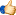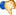## General Questions >> General Question

### Closed Question

• How do you calculate your GPA?

A=4.0
B=3.0
C=2.0
D=1.0
F=0.0

90-100 = A
80-89 = B
70-79 = C
60-69 = D
<60 = F

Depending on the range, assign A, B, C, D or F to each class you are taking and then match the corresponding GPA with it. Add up all the numbers and then divide that by the number of classes you are taking. That will give you your GPA.

• 0• 0•   Report It